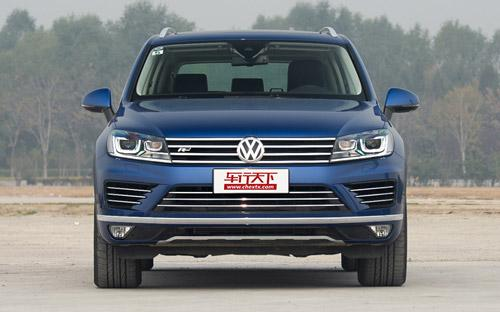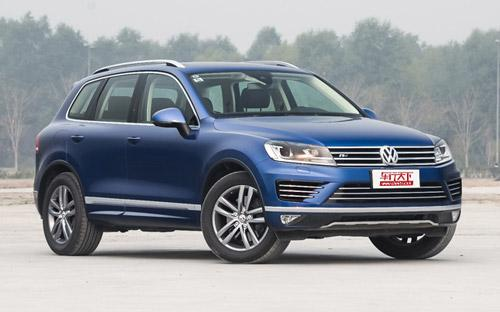## 大众(进口) 途锐11 种颜色可选2016款最低售价：73.90 万元起

4801(mm)1940(mm)1709(mm)##### 配置亮点：
• 胎压监测装置

• ISOFIX儿童座椅接口

• 车身稳定控制(ESC/ESP/DSC等)

• 电动天窗

• 定速巡航

• 后倒车雷达

• 真皮座椅

• GPS导航系统

• 氙气大灯

• 后视镜加热

2016款 3.0TSI 耀越版 (257张)
• 2016款 3.0TSI 耀越版 (257张)
• 2016款 3.0TSI 高配型 (172张)
• 2016款 3.0TSI R-Line (90张)
• 2016款 3.0TSI 标配型 (149张)大众(进口) 途锐 在售车型

排量 车型 厂商指导价 本地最低报价 购车工具
3.0L 3.0TSI R-Line 8挡手自一体 94.60万 询底价+对比
4.2L 3.0TSI R-Line 8挡手自一体 94.60万 询底价+对比
3.0T 3.0TSI 标配型 8挡手自一体 73.90万 询底价+对比
3.0TSI 耀越版 8挡手自一体 76.90万 询底价+对比
3.0TSI 高配型 8挡手自一体 83.80万 询底价+对比

大众(进口) 途锐 经销商

查看更多 >>
更多 >>

本地降价排名

### 大众(进口) 途锐 动力加速

途锐 0-100公里加速时间分布在 6.5-7.6秒 属于 高性能级

动力级别 加速时间 车型
高性能级(2款) 7.0s 3.0TSI R-Line3.0TSI 标配型3.0TSI 耀越版3.0TSI 高配型
6.5s 4.2L V8
运动级(2款) 7.1s 3.0TSI V6 R-Line 豪华型3.0TSI V6 标配型3.0TSI V6 舒适型3.0TSI V6 高配型3.0TSI V6 豪华型3.0TSI V6 耀锐限量版3.0TSI V6 黑色探险者3.0TSI V6 新锐版
7.6s 3.0TDI V6 柴油标配型3.0TDI V6 柴油舒适型

大众(进口) 途锐 视频

大众(进口) 途锐 新闻资讯

# 新款大众途锐正式上市 售价69.5-92.5万元

进口新车 超过9322次关注

据官方获悉，2015款途锐已经在国内正式上市，新车保留了现款车型的设计，仅仅是在部分细节上有所改变；搭载排量为3.0L的柴油或汽油发动机，共有7款车型，售价区间为...

# 新款途锐搭新3.0TDI发动机 提升22马力

技术 超过5783次关注

日前，大众汽车官方称，旗下新款途锐将换装全新的3.0TDI发动机，这台发动机的最大输出功率比老发动机提升22马力。除此之外，今年10月开始，海外新款途锐将可提供空...

# 实拍2015款途锐R-Line 变化集中在外观

评测 超过4952次关注

途锐作为大众旗下的中大型SUV，以相对实在的价格近年来获得了不少国内消费者的青睐。在2014北京车展上，它的2015款车型正式发布，新车主要针对外观进行了改动，部分...

# 实拍大众途锐4.2L V8 挑起车系旗舰重任

评测 超过5691次关注

途锐作为一款豪华SUV，其低调的外形和不错的性价比，一直是吸引消费者关注的两大亮点。这一代途锐最早仅有3.0T车型，之后又推出了3.6L车型，而4.2LV8车型，在2013广...

新闻 超过0次关注

# 大众新款途锐官图发布 北京车展发布

新闻 超过5690次关注

日前，大众发布了新款途锐的官图，新车在外观和内饰方面都有所变化。这款车将会在北京车展上发布。

# 新款途锐搭新3.0TDI发动机 配置可选装

进口新车 超过5813次关注

日前，大众汽车官方称，旗下新款途锐将换装全新的3.0TDI发动机，这台发动机的最大输出功率比老发动机提升22马力。除此之外，今年10月开始，海外新款途锐将可提供空...

猜你喜欢

﻿
• 快速找车
• 选择品牌
• 选择品牌
• A  奥迪
• A  阿斯顿·马丁
• A  阿尔法·罗密欧
• B  宝沃
• B  布加迪
• B  巴博斯
• B  保时捷
• B  宾利
• B  奔驰
• B  宝马
• B  本田
• B  别克
• B  标致
• B  比亚迪
• B  宝骏
• B  北汽制造
• B  北汽新能源
• B  北汽幻速
• B  北汽威旺
• B  北京汽车
• B  奔腾
• B  北汽绅宝
• C  长安
• C  长安商用
• C  长城
• C  昌河
• D  大众
• D  道奇
• D  DS
• D  东南
• D  东风风神
• D  东风风行
• D  东风小康
• D  东风风度
• D  东风
• F  福特
• F  丰田
• F  菲亚特
• F  法拉利
• F  福田
• F  福迪
• F  福汽启腾
• G  观致
• G  广汽传祺
• G  广汽吉奥
• G  GMC
• H  红旗
• H  汉腾汽车
• H  哈弗
• H  哈飞
• H  海格
• H  海马
• H  华颂
• H  黄海
• H  华泰
• H  恒天
• J  吉利汽车
• J  捷豹
• J  Jeep
• J  江淮
• J  江铃
• J  金杯
• J  九龙
• J  金旅
• K  凯翼
• K  凯迪拉克
• K  克莱斯勒
• K  科尼塞克
• K  卡威
• K  开瑞
• L  路虎
• L  林肯
• L  劳斯莱斯
• L  兰博基尼
• L  雷克萨斯
• L  铃木
• L  雷诺
• L  理念
• L  力帆
• L  莲花汽车
• L  猎豹
• L  路特斯
• L  陆风
• M  马自达
• M  MG
• M  MINI
• M  玛莎拉蒂
• M  摩根
• M  迈凯轮
• N  纳智捷
• O  欧宝
• O  讴歌
• O  欧朗
• Q  奇瑞
• Q  起亚
• Q  启辰
• R  日产
• R  荣威
• R  瑞麒
• S  三菱
• S  斯威汽车
• S  萨博
• S  smart
• S  斯柯达
• S  斯巴鲁
• S  思铭
• S  双龙
• S  上汽大通
• S  双环
• T  特斯拉
• T  腾势
• W  沃尔沃
• W  五菱汽车
• W  五十铃
• W  威兹曼
• W  威麟
• X  现代
• X  雪佛兰
• X  雪铁龙
• X  西雅特
• Y  一汽
• Y  英菲尼迪
• Y  英致
• Y  依维柯
• Y  野马汽车
• Y  永源
• Z  众泰
• Z  中华
• Z  中兴
• Z  知豆
• 选择车系
• 选择车系
• 车型对比
• 选择品牌
• 选择品牌
• A  奥迪
• A  阿斯顿·马丁
• A  阿尔法·罗密欧
• B  宝沃
• B  布加迪
• B  巴博斯
• B  保时捷
• B  宾利
• B  奔驰
• B  宝马
• B  本田
• B  别克
• B  标致
• B  比亚迪
• B  宝骏
• B  北汽制造
• B  北汽新能源
• B  北汽幻速
• B  北汽威旺
• B  北京汽车
• B  奔腾
• B  北汽绅宝
• C  长安
• C  长安商用
• C  长城
• C  昌河
• D  大众
• D  道奇
• D  DS
• D  东南
• D  东风风神
• D  东风风行
• D  东风小康
• D  东风风度
• D  东风
• F  福特
• F  丰田
• F  菲亚特
• F  法拉利
• F  福田
• F  福迪
• F  福汽启腾
• G  观致
• G  广汽传祺
• G  广汽吉奥
• G  GMC
• H  红旗
• H  汉腾汽车
• H  哈弗
• H  哈飞
• H  海格
• H  海马
• H  华颂
• H  黄海
• H  华泰
• H  恒天
• J  吉利汽车
• J  捷豹
• J  Jeep
• J  江淮
• J  江铃
• J  金杯
• J  九龙
• J  金旅
• K  凯翼
• K  凯迪拉克
• K  克莱斯勒
• K  科尼塞克
• K  卡威
• K  开瑞
• L  路虎
• L  林肯
• L  劳斯莱斯
• L  兰博基尼
• L  雷克萨斯
• L  铃木
• L  雷诺
• L  理念
• L  力帆
• L  莲花汽车
• L  猎豹
• L  路特斯
• L  陆风
• M  马自达
• M  MG
• M  MINI
• M  玛莎拉蒂
• M  摩根
• M  迈凯轮
• N  纳智捷
• O  欧宝
• O  讴歌
• O  欧朗
• Q  奇瑞
• Q  起亚
• Q  启辰
• R  日产
• R  荣威
• R  瑞麒
• S  三菱
• S  斯威汽车
• S  萨博
• S  smart
• S  斯柯达
• S  斯巴鲁
• S  思铭
• S  双龙
• S  上汽大通
• S  双环
• T  特斯拉
• T  腾势
• W  沃尔沃
• W  五菱汽车
• W  五十铃
• W  威兹曼
• W  威麟
• X  现代
• X  雪佛兰
• X  雪铁龙
• X  西雅特
• Y  一汽
• Y  英菲尼迪
• Y  英致
• Y  依维柯
• Y  野马汽车
• Y  永源
• Z  众泰
• Z  中华
• Z  中兴
• Z  知豆
• 选择车系
• 选择车系
• 选择车型
• 选择车型
• 意见反馈